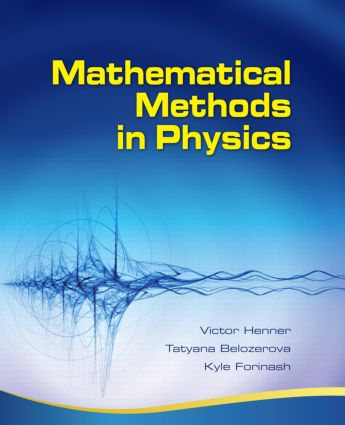Mathematical Methods in Physics

Partial Differential Equations, Fourier Series, and Special Functions, 1st Edition

A K Peters/CRC Press

860 pages

Purchasing Options:\$ = USD
Hardback: 9781568813356
pub: 2009-06-18
SAVE ~\$29.00
Currently out of stock
\$145.00
\$116.00
x
eBook (VitalSource) : 9780429062520
pub: 2009-06-18
from \$72.50

FREE Standard Shipping!

Description

This book is a text on partial differential equations (PDEs) of mathematical physics and boundary value problems, trigonometric Fourier series, and special functions. This is the core content of many courses in the fields of engineering, physics, mathematics, and applied mathematics. The accompanying software provides a laboratory environment that allows the user to generate and model different physical situations and learn by experimentation. From this standpoint, the book along with the software can also be used as a reference book on PDEs, Fourier series and special functions for students and professionals alike.

Reviews

In comparison with typical introductions to partial differential equations, the book and attached software are significantly more detailed. It explains various examples of physical problems and solves related partial differential equations under different types of boundary conditions. The authors do more with special functions and carry out examples of Fourier analysis using these functions. The book, along with the software, can also be considered as a reference book on PDEs, Fourier series and some of the special functions for students and professionals. As a text, this book can be used in an advanced course on mathematical physics (or related courses) for advanced students of engineering, physics, mathematics, and applied mathematics.

—Soheila Emamyari and Mehdi Hassani, MAA Reviews, November 2009

[Henner, Forinash, and Belozerova] address the main topics of many courses in mathematical physics within the fields of engineering, physics, mathematics, and applied mathematics. The texbook and accompanying software are significantly more detailed than typical introductions to partial differential equations, they say, and provide examples on setting up physical problems as mathematical ones, solving partial differential equations under different types of boundary conditions, working with special functions, and carrying out a Fourier analysis using these functions. The software provides a simple interface, and does not require students to learn a programming language.

Book News Inc., September 2009

Fourier Series

Periodic Processes and Periodic Functions

Fourier Formulas

Orthogonal Systems of Functions

Convergence of Fourier Series

Fourier Series for Non-periodic Functions

Fourier Expansions on Intervals of Arbitrary Length

Fourier Series in Cosine or Sine Functions

The Complex Form of the Fourier Series

Complex Generalized Fourier Series

Fourier Series for Functions of Several Variables

Uniform Convergence of Fourier Series

The Gibbs Phenomenon

Completeness of a System of Trigonometric Functions

General Systems of Functions: Parseval’s Equality and Completeness

Approximation of Functions in the Mean

Fourier Series of Functions Given at Discrete Points

Solution of Differential Equations by Using Fourier Series

Fourier Transforms

The Fourier Integral

Problems

Sturm-Liouville Theory

The Sturm-Liouville Problem

Mixed Boundary Conditions

Examples of Sturm-Liouville Problems

Problems

One-Dimensional Hyperbolic Equations

Derivation of the Basic Equations

Boundary and Initial Conditions

Other Boundary Value Problems: Longitudinal Vibrations of a Thin Rod

Torsional Oscillations of an Elastic Cylinder

Acoustic Waves

Waves in a Shallow Channel

Electrical Oscillations in a Circuit

Traveling Waves: D’Alembert Method

Semi-infinite String Oscillations and the Use of Symmetry Properties

Finite Intervals: The Fourier Method for One-Dimensional Wave Equations

Generalized Fourier Solutions

Energy of the String

Problems

Two-Dimensional Hyperbolic Equations

Derivation of the Equations of Motion

Oscillations of a Rectangular Membrane

The FourierMethod Applied to Small Transverse Oscillations of a Circular Membrane

Problems

One-Dimensional Parabolic Equations

Physical Problems Described by Parabolic Equations: Boundary Value Problems

The Principle of the Maximum, Correctness, and the Generalized Solution

The Fourier Method of Separation of Variables for the Heat Conduction Equation

Heat Conduction in an Infinite Bar

Heat Equation for a Semi-infinite Bar

Problems

Parabolic Equations for Higher-Dimensional Problems

Heat Conduction in More than One Dimension

Heat Conduction within a Finite Rectangular Domain

Heat Conduction within a Circular Domain

Problems

Elliptic Equations

Elliptic Partial Differential Equations and Related Physical Problems

The Dirichlet Boundary Value Problem for Laplace’s Equation in a Rectangular Domain

Laplace’s and Poisson’s Equations for Two-Dimensional Domains with Circular Symmetry

Laplace’s Equation in Cylindrical Coordinates

Problems

Bessel Functions

Boundary Value Problems Leading to Bessel Functions

Bessel Functions of the First Kind

Properties of Bessel Functions of the First Kind: Jn (x)

Bessel Functions of the Second Kind

Bessel Functions of the Third Kind

Modified Bessel Functions

The Effect of Boundaries on Bessel Functions

Orthogonality and Normalization of Bessel Functions

The Fourier-Bessel Series

Further Examples of Fourier-Bessel Series Expansions

Spherical Bessel Functions

The Gamma Function

Problems

Legendre Functions

Boundary Value Problems Leading to Legendre Polynomials

Generating Function for Legendre Polynomials

Recurrence Relations

Orthogonality of Legendre Polynomials

The Multipole Expansion in Electrostatics

Associated Legendre Functions P m (x)

n

Orthogonality and the Normof Associated Legendre Functions

Fourier-Legendre Series in Legendre Polynomials

Fourier-Legendre Series in Associated Legendre Functions

Laplace’s Equation in Spherical Coordinates and Spherical Functions

Problems

Eigenvalues and Eigenfunctions of the Sturm-Liouville Problem

Auxiliary Functions for Different Types of Boundary Conditions

The Sturm-Liouville Problem and the Laplace Equation

Vector Calculus

How to Use the Software Associated with this Book

Program Overview

Examples Using the Program TrigSeries

Examples Using the Program Waves

Examples Using the Program Heat

Examples Using the Program Laplace

Examples Using the Program FourierSeries

Victor Henner, Department of Physics and Astronomy, University of Louisville, Kentucky, USA

Tatyana Belozerova, Perm State University, Russia

Kyle Forinash, Professor of Physics and Program Coordinator, Indiana University Southeast, New Albany, USA